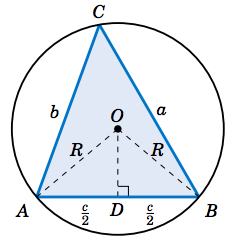# 多邊形外接圓

the intersection of the perpendicular bisectors of any two sides of the polygon三角形邊角關係 (the side-angle relationships) 的定性描述 (qualitative descriptions) 為

• 大邊對大角，小邊對小角
In any triangle, the greater side subtends the greater angle.
• 大角對大邊，小角對小邊
In any triangle, the greater angle is subtended by the greater side.

The ratio of a side of a triangle to the sine of its opposite angle is a constant. The constant is the diameter of the triangle's circumcircle, or $$2R$$ where $$R$$ is the circumradius.

 [語音講解：circumcirc.mp3]

[ 回上層 ]

Created: July 20, 2022
Last Revised: 07/26, 11/14